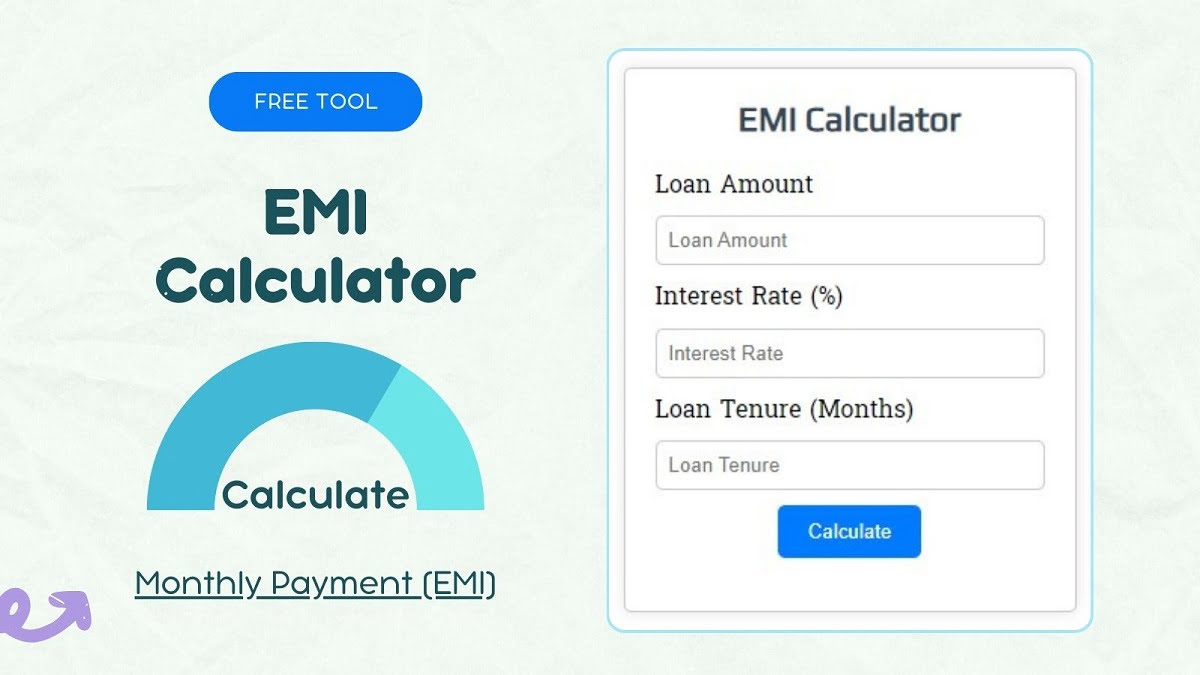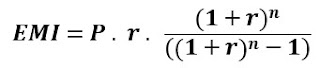# EMI Calculator Nepal: Calculate Monthly Payment for Loan in Nepal

## What is EMI Calculator Nepal?

An EMI Calculator Nepal is a tool used to calculate Equated Monthly Installments (EMIs) for loans or mortgages. It helps individuals estimate the amount they need to pay each month to repay their loan, taking into account the principal amount, interest rate, and loan tenure. This tool is commonly used for various types of loans, such as personal loans, home loans, and car loans, to better understand the financial commitment involved in borrowing.## How to work EMI Calculator Nepal?

An EMI (Equated Monthly Installment) calculator in Nepal works by taking three key inputs: the loan amount, the interest rate, and the loan tenure. Using these inputs, it calculates the fixed monthly payment that a borrower needs to make in order to repay the loan over the specified duration. Here's how an EMI calculator typically works:

#### Input Collection

• Loan Amount: The total amount borrowed from the lender.
• Interest Rate: The annual interest rate (expressed as a percentage) charged by the lender.
• Loan Tenure: The duration of the loan repayment in months.

#### Interest Calculation

• Convert the annual interest rate into a monthly interest rate by dividing it by 12 (the number of months in a year) and then converting it into a decimal.
• For example, if the annual interest rate is 36%, the monthly interest rate would be (36 / 12) / 100 = 0.03.

#### EMI Calculation

• The Monthly Payment EMI is calculated using the formula for the monthly installment of an amortizing loan:#### Where,

• 'P' is the principal loan amount (initial loan amount).
• 'r' is the monthly interest rate.
• 'n' is the total number of monthly installments (loan tenure in months).

#### Display Result

• The calculated Monthly Payment (EMI) is displayed to the user as the fixed amount they need to pay each month.

• Many EMI calculators also provide additional information, such as the total interest paid over the loan tenure and the total repayment amount (principal + interest).

You can input different combinations of loan amount, interest rate, and tenure to see how it affects your monthly payments. EMI calculators in Nepal are widely used by borrowers to understand the financial commitment involved in taking a loan and to plan your budgets accordingly.

1.1.